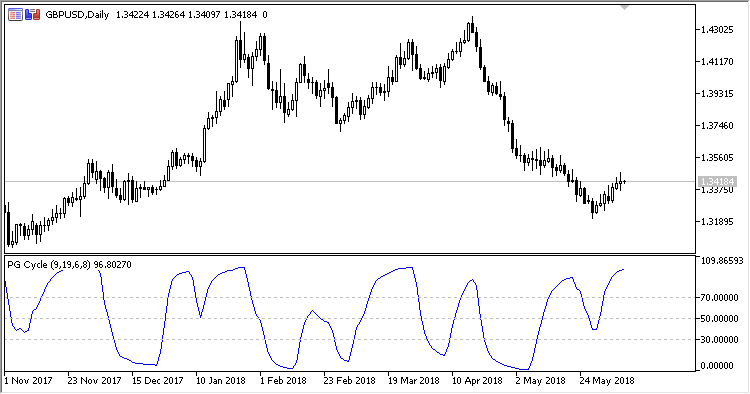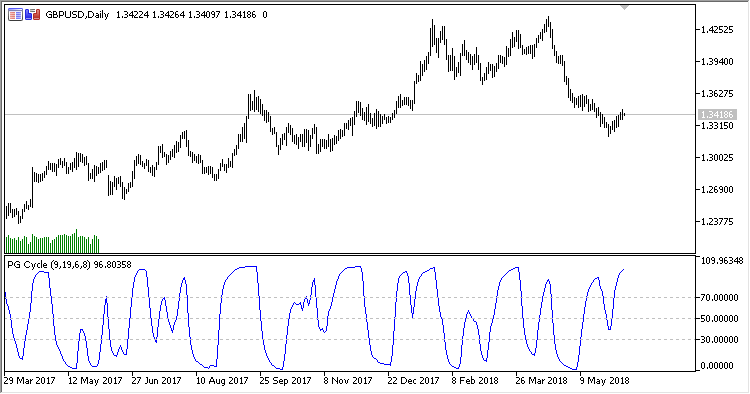Interesting script?
So post a link to it -
let others appraise it

You liked the script? Try it in the MetaTrader 5 terminal# PGC - indicator for MetaTrader 5

Views:
855
Rating:
Published:
2018.07.09 13:31

Oscillator indicating the cyclic changes in the market.

• First MA period - first EMA period;
• Second MA period - second EMA period;
• Smoothing period - smoothing period;
• RSI period - RSI calculation period;
• Applied price - the calculation price;
• Overbought level - overbought level;
• Oversold level.

Calculation:

PGC =100.0 - 100.0 / (1.0+RS)

where:

```if AvgDec != 0
RS = AvgInc / AvgDec
otherwise
RS = 0
AvgDec = EMA(Dec, RSI Period)
AvgInc = EMA(Inc, RSI Period)
If Z3 > PrevZ3
Inc = Z3 - PrevZ3, Dec = 0
If Z3 < PrevZ3
Dec = PrevZ3 - Z3, Inc = 0
```
Z3 = 2.0 * AvgMA3 - Avg2MA3
```Avg2MA3 = EMA(AvgMA3, Smoothing period)
AvgMA3 = EMA((Z1 - Z2), Smoothing period)
Z2 = 2.0 * MA2 - AvgMA2
Z1 = 2.0 * MA1 - AvgMA1
AvgMA2 = EMA(MA2, First MA period)
AvgMA1 = EMA(MA1, First MA period)
MA2 = EMA(Applied price, Second MA period)
MA1 = EMA(Applied price, First MA period)
```Translated from Russian by MetaQuotes Software Corp.
Original code: https://www.mql5.com/ru/code/21047Reverse_Engineering_RSI

the indicator performs an inverse mathematical transform of oscillator RSI into price and helps assume, at which approximate level the close price of the next day will be, if RSI reaches a certain value.VAMA_MACDExp_UltraAbsolutelyNoLagLwmaVolume trader